# 了解功率电感参数## 电感是什么？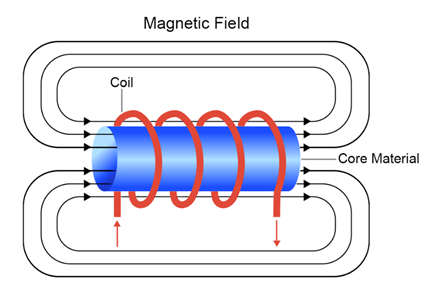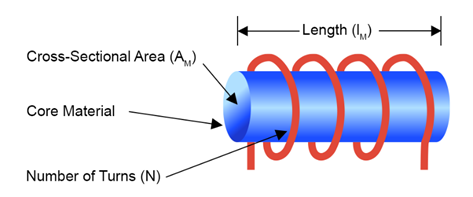公式 参数 参数描述 $$L = \frac {µ_r µ_0 \times A_M}{I_M} \times N^2$$ µ = µr µ0 磁导率 µr 相对磁导率（磁芯） µ0 = 4π10-7 磁场常数（真空磁导率） AM 线圈面积（磁场面积） IM 线圈长度（磁场长度） µ 匝数

## 磁导率

 公式 参数 参数描述 $$B = µ \times H$$ $$µ$$ 介质的磁导率 $$H$$ 磁场（取决于几何形状、匝数和电流）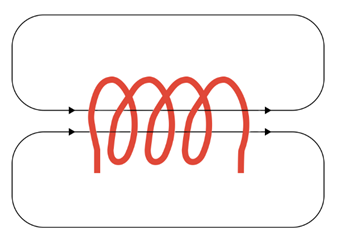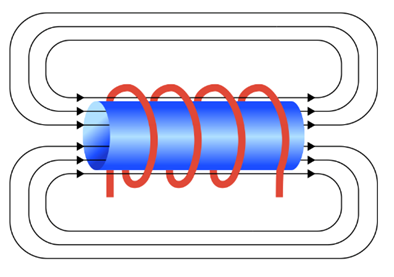芯材 符号 磁导率 铁 µr FEBASED 50 至 150 镍锌 µr NiZn 40 至 1,500 锰锌 µr MnZn 300 至 20,000

## 电感值 (L)

 公式 参数 参数说明 $$v = L \times \frac {di}{dt}$$ $$v$$ 电感两端的压降 $$\frac {di}{dt}$$ 电流变化率

## 电阻 (R)

 公式 参数 参数说明 $$R_{DC} = ρ \times \frac {I}{A}$$ $$ρ$$ 电阻率 $$I$$ 长度 $$A$$ 横截面面积

 尺寸 线的类型 比较 横截面积 直径1mm 圆线$$A_{ROUND} = πr^2 = π \times 0.5^2 = 0.785mm^2$$ 1mm x 1mm (宽/厚) 扁线$$A_{FLAT} = 1 \times 1 = 1mm^2$$

 圆线 扁线 电感值更高 电阻(RDC)更高 横截面较小 匝数可以更多 电流较小 绕组面积有限，降低了最大电感值 电阻(RDC)较低 绕组窗口可以被完全利用 匝数较少 电流更高

$$P_{DC} = I^2_{DC} \times R_{DC}$$

(PAC)铜损则取决于 RAC，它是由频率驱动的邻近效应和趋肤效应引起的。频率越高，PAC 铜损越高。

## 纹波电流 (∆IL)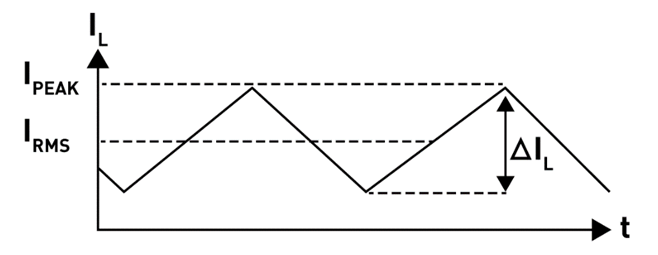## 额定电流 (IDC, IRMS)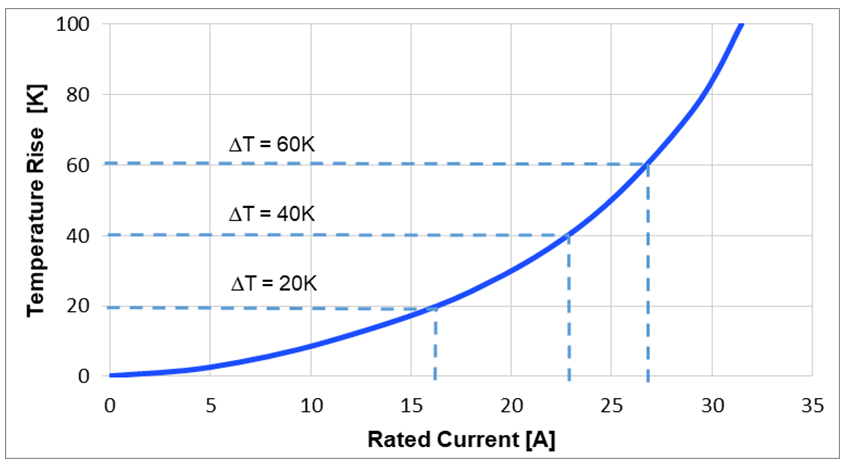$$T_{OP} = T_{AMB} + ΔT$$

## 饱和电流 (ISAT)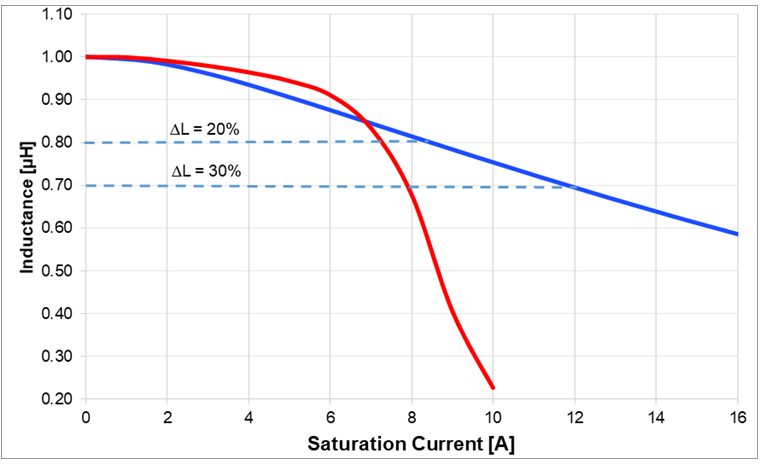## 自谐振频率和阻抗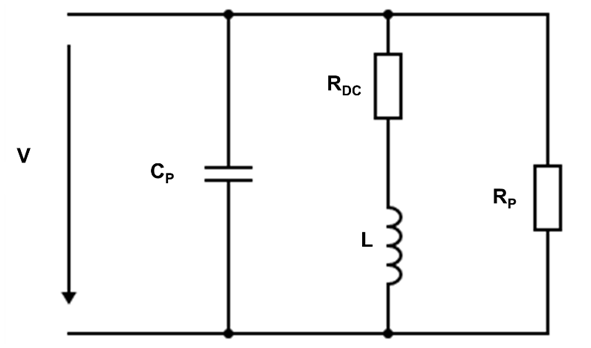$$X_L = X_C \to jωL = \frac {1}{jωC}$$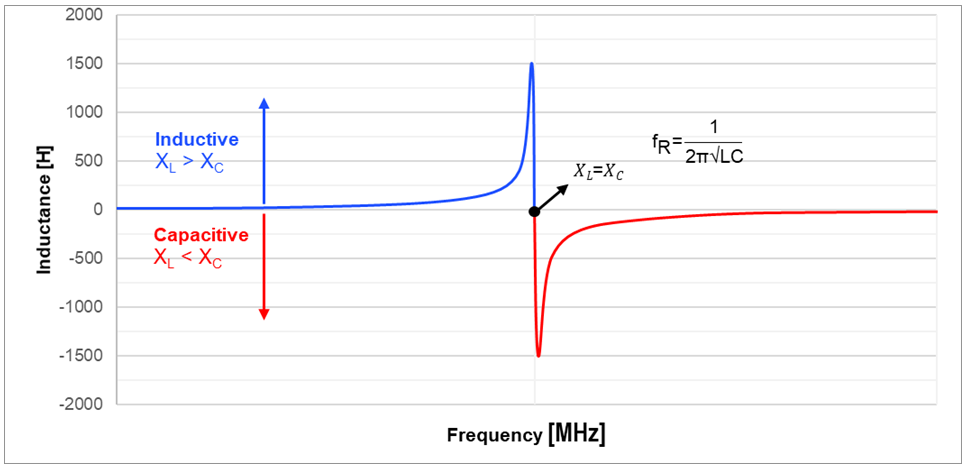## 选择具有高性价比的紧凑型电感

MPS 可以提供各种功率电感，适用于电源到电源变换器的各种应用。尤其是 MPL-SE 半屏蔽式电感系列，其外部使用电磁环氧树脂材料，具有更好的导磁特性。

MPS 的电感和 DC/DC 电源变换器产品将为您的设计提供简单而完善的电源解决方案。